# RBSE Solutions For Class 12 Physics Chapter 8: Magnetism and Properties of Magnetic Substances | Textbook Important Questions & Answers

The answers are provided for all the questions of Chapter 8 Physics of RBSE Class 12. Students can go through these questions to understand the concepts better and score well in the board examination and entrance examinations for various professional courses. These solutions are provided by the team of experts and offer the best and accurate solutions to the questions. Do check BYJU’S RBSE Class 12 solutions page to get the answers for textbook questions of all subjects.

## Multiple Choice Questions

Q1: If the distance between two magnetic poles of unit magnetic strength is 1m, then the force acting between them will be

1. 4π x 10-7 N
2. 4π N
3. 10-7 N
4. 4π /10-7 N

Q2: Magnetic susceptibility for superconductors is

1. +1
2. -1
3. Zero
4. infinity

Q3: Magnetic susceptibility of free space is

(a) +1

(b) -1

(c) Zero

(d) infinity

Q4: Magnetic susceptibility is negative and very less for

1. Ferromagnetic substances
2. Paramagnetic substances
3. Diamagnetic substances
4. All the above

Q5: If the relative permeability of a substance is 1.00001, then substances will be

1. Ferromagnetic
2. Paramagnetic
3. Diamagnetic
4. None of these

Q1: A magnetic needle is free to rotate in the vertical plane about the horizontal axis. What direction will it indicate at magnetic poles?

Answer: The free magnetic needle orients itself with its axis vertical. Therefore, the magnetic field will be in the vertical direction and the horizontal component of earth’s magnetic field is zero.

Q2: Name the type of magnetic material which does not depend on temperature?

Diamagnetic substances do not depend on the temperature

Q3: How the value of dip angle changes in going from equator to poles?

From going from equator to poles the dip angle increases from 0 degrees to 90 degrees

Q4: A magnetic material has magnetic susceptibility as -0.085. What type of material is it?

The type of material is diamagnetic.

Q5: What is retentivity or remanence?

After removing the magnetic field from the sample, some magnetic flux density is left behind in the sample and this is called retentivity or remanence.

Q6: Name two paramagnetic substances.

(i)CuCl2

(ii)Oxygen

Q7: What is a magnetic meridian?

Magnetic meridian is the imaginary line connecting the magnetic south and magnetic north poles. The earth’s magnetic field acts in the direction of the magnetic meridian.

Q8: Where on earth’s surface is the dip angle zero and 90 degrees?

Answer: The dip angle is zero at the magnetic equatorial line and it is zero at the pole.

Q9: Write the relation between magnetic permeability and magnetic susceptibility for a medium.

μr= (1+Xm)

μr is the magnetic permeability

Xm is the magnetic susceptibility

Q10: Write the unit of pole strength.

Answer: The unit of magnetic pole strength is Ampere metre (A.m)

Q11: What will be the value of dip angle, where the ratio of the vertical and horizontal component of earth’s magnetic field is 1/

Bv/BH = 1/

We know

Bv/BH=tan θ

Therefore, we have

tan θ = 1/

tan θ = tan 300

θ = 300

Q12: What is magnetic hysteresis?

Answer: The lagging of magnetic induction behind the magnetising field when the magnetic material is subjected to a cycle of magnetisation is called magnetic hysteresis.

Q13: What will be the ratio of magnetic fields at the points on-axis and equator, equidistant from the centre of a bar magnet?

The magnetic field on the axial line is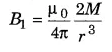The magnetic field on the equatorial line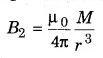B1/B2= 2/1

Therefore, the ratio is given by

B1: B2 = 2:1

Q14: What will be the value of dip angle at a place where vertical and horizontal components of earth’s magnetic field are equal?

Bh is the horizontal component = Bcosx

Bv is the vertical component = Bsinx

x=tan-1 (Bv/BH)

Since it is given Bv = Bh

x = tan-1 (1) 450

The angle of dip is 450

Q15: What will be the change in the magnetic moment of bar magnetic if we bisected it along its length?

The magnetic moment of a bar magnet of length L is with pole strength m is

M=m x l

If the bar magnet is divided into 2. The length becomes l/2. Therefore, the magnetic moment will be

M1 = mx(l/2) = ml/2 = M/2

Q1: Find the expression for the potential energy of a bar magnet placed in the uniform magnetic field B, such that the angle between its dipole moment and B is θ.

The work done in rotating a dipole against the action of torque is stored as potential energy.

The expression for torque is

τ = MBsinθ

Where M is the dipole moment and B is the strength of the magnetic field

The work done in rotating the dipole through an angle dθ is

dW = τdθ = MBsinθdθ

If the dipole is rotated from the initial position θ=θ1 to θ=θ2. The work done is given as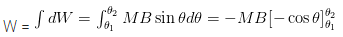W = -MB(cosθ2 – cosθ1)

This work done is stored as potential energy

U = -MB(cosθ2 – cosθ1)

Q2: How will you identify rods of paramagnetic and diamagnetic substances?

The rods made of paramagnetic material will set parallel to the field and the rods of diamagnetic material will be perpendicular to the field.

Q3: Why do we get two null points for a bar magnet? Can we get only one null point? How?

Null points are the point where the magnetic field due to the magnet is equal and opposite to the horizontal component of the earth’s magnetic field. The resultant magnetic field at these points will be zero. In this way, two null points are obtained for a bar magnet.

When the bar magnet is kept vertically only one null point is obtained.

Q4: Why is soft iron used in making electromagnet?

Answer: Soft iron has high permeability and low retentivity and coercivity. Therefore, it is used in making an electromagnet.

Q5: A bar magnet of the magnetic moment M is placed parallel to a uniform magnetic field B. What will be the work done in rotating it by 90 degrees.

Given θ1=0 degrees

θ2= 90 degrees

The work done is given by

$$\begin{array}{l}W=MB(cos\theta _{1} – cos\theta _{2})\end{array}$$

W= MB(Cos0 – Cos 90)

W= MB(1-0)

W=MB

Q6: Define angle of declination and dip angle.

Angle of declination: The angle between the geometric meridian and the magnetic meridian at a place is called the magnetic declination at that place.

Angle of dip: The angle made by the earth’s total magnetic field with the horizontal direction in the magnetic meridian is called the angle of dip.

Q7: Write down the Curie-Wies Law and write the value of curie temperature for iron.

Curie-Wies Law: Above the Curie point (paramagnetic phase) the susceptibility varies with temperature as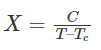Where C= material-specific constant

T= Absolute Temperature

Tc = Curie temperature

Curie temperature of iron is 1043 K

Q8: Write four properties of the magnetic field lines.

1. Outside the magnet, magnetic field lines emerge from the north pole and merge at the south pole.
2. As the distance between the poles increases, the density of magnetic lines decreases.
3. The direction of field lines inside the magnet is from the South Pole to the North Pole.
4. Magnetic lines do not intersect with each other.
5. As the number of magnetic field lines crossing per unit area increases, the stronger is the magnitude of the magnetic field.

Q9: What is the behaviour of diamagnetic, paramagnetic and ferromagnetic substances in non-uniform magnetic field

1. A diamagnetic substance moves from the stronger to the weaker parts of the field when placed in a non-uniform magnetic field.
2. A paramagnetic substance moves from the weaker to the stronger parts of the field when placed in a non-uniform magnetic field.
3. A ferromagnetic substance moves from the weaker to the stronger parts of the field when placed in a non-uniform magnetic field.

Q10: What is Gauss’s Law for magnetism? What does it indicate?

Gauss’s Law for magnetism: The law states that the magnetic field over a closed surface S is equal to zero.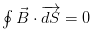Gauss’s Law for magnetism indicates that no magnetic monopoles exist and total flux through a closed surface is zero.

Q11: Why are magnetic field lines closed loops?

Answer: The magnetic field lines form closed loops because monopoles do not exist in nature. The direction of the magnetic field lines outside the magnet is from the North pole to the South Pole and inside the magnet is from South to North. Therefore, they form closed loops.

Q12: Compare magnetic fields of a bar magnet and a current-carrying solenoid.

Similarities of magnetic fields and bar magnet

1. Bar magnet and solenoid both have attractive and directive properties, ie, to align itself along the external magnetic field.
2. Both have two poles
3. They attract magnetic substances
4. The magnetic field at the axial point is the same for both.
5. The magnetic moment is the same for both.

Differences between magnetic fields and bar magnet

1. The bar magnet is a permanent magnet whereas a solenoid is an electromagnet i.e., it acts as a magnet only when an electric current is passed through.
2. When a bar magnet is cut into two halves, both the pieces act as a magnet with the same magnetic properties whereas when a solenoid is cut into two halves, they will have weaker fields.
3. The poles of the bar magnet are fixed whereas for a solenoid the poles can be altered.
4. The strength of the magnetic field of a bar magnet is fixed i.e., unaltered whereas the strength of the magnetic field of a solenoid depends on the electric current that is passed through it.

Q13: What is the cause of Earth’s magnetism?

The Earth’s magnetism is caused by the convection current of molten iron and nickel in the earth’s core. These currents carry streams of charged particles and generate magnetic fields. This magnetic field deflects ionising charged particles coming from the sun (called solar wind) and prevents them from entering our atmosphere.

Q14: What are the uses of the hysteresis curve?

1. The hysteresis curve provides information about the magnetic properties of the material and in selecting suitable devices for electric material.
2. The residual magnetism can be calculated from the hysteresis curve. Therefore, it is simple to choose material for electromagnets.
3. The value of retentivity and coercivity can be found using the hysteresis curve. These values help in selecting the right material as a permanent magnet.

Q15: Find the expression for torque on a bar magnet placed at an angle with the uniform magnetic field. When will it be maximum?

Consider a bar magnet NS of length l in a uniform magnetic field. The bar magnet makes an angle Ө with the magnetic field.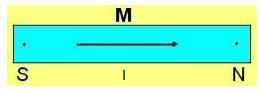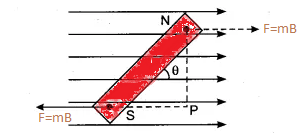Force on the north pole = mB, along the direction of the magnetic field

Force on the south pole = mB, opposite to the direction of the magnetic field

The force acting on the two poles are equal but opposite in direction.

The Torque τ is given by

τ= force x Perpendicular distance from the axis of the bar magnet.

τ= mB xlsinθ

τ=(ml)Bsinθ

τ = MBsinθ (here M=ml is the magnetic dipole moment)

When the bar magnet is perpendicular to the magnetic field (θ=900)

Therefore τ = MBsin900

τ = MB

The torque is maximum when the bar magnet is perpendicular.

## Essay Type Questions

1) What are the elements of the earth’s magnetism? Define them and show with a labelled diagram.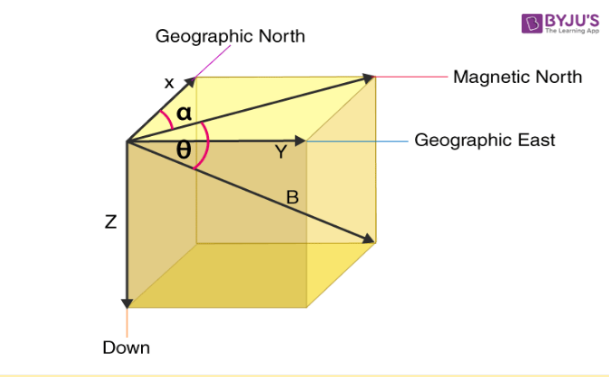Magnetic Declination

Magnetic Declination is defined as the angle between the geographic north and the magnetic north. In the figure above, the angle of declination is represented as α. On the horizontal plane, the geographic north is never at a constant position and keeps varying depending upon the position on the earth’s surface and time.

Angle of dip

The magnetic inclination is also known as the angle of dip. It is the angle made by the horizontal plane on the earth’s surface. At the magnetic equator, the angle of dip is 0° and at the magnetic poles, the angle of dip is 90°. In the figure above the angle of dip is the angle θ

Horizontal Component of Earth’s Magnetic field

If the direction of the earth’s magnetic field is in the horizontal direction in the magnetic meridian If θ is the angle of dip then the earth’s magnetic field at a place is given by

Horizontal component BH = Bcosθ

Vertical component BV= Bsinθ

tan θ =BV/BH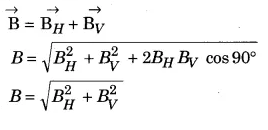Q2: Explain diamagnetism, discuss its properties. Write a few difference between paramagnetic and diamagnetic substances.

Diamagnetism

These materials have paired electrons in the atoms, which revolve in opposite directions. When there is no electric field the magnetic moment of these electrons gets cancelled. In the presence of a magnetic field, the force on the electrons is in opposite directions. On one electron it is towards the nucleus on the other electron it is away from the nucleus. The magnetic moment in the direction of the field gets decreased and that which is in the opposite direction increases. Hence, the net magnetism in the material is opposite to the applied field.

Properties of Diamagnetic Materials

1. There are no atomic dipoles in diamagnetic materials because the resultant magnetic moment of each atom is zero due to paired electrons.

2. Diamagnetic materials are repelled by a magnet.

3. The substances are weakly repelled by the field so in a nonuniform field, these substances have a tendency to move from a strong to a weak part of the external magnetic field.

4. The intensity of magnetization I is very small, negative and proportional to the magnetizing field.

5. Magnetic susceptibility is small and negative.

6. The relative permeability is slightly less than unity.

7. These materials are independent of temperature, diamagnetic materials do not obey Curie’s law.

8. A rod of diamagnetic material comes to rest with its length perpendicular to the direction of the field when it is suspended in a uniform magnetic field because the field is strongest at the poles.

Difference between paramagnetic and diamagnetic substances

 Paramagnetic substances Diamagnetic substances They get weakly magnetised in an external magnetic field They get strongly magnetised in an external magnetic field Paramagnetic substances have at least one unpaired electron All the electrons are paired The magnetic field created is in the direction of the magnetic field The magnetic field created is in the direction opposite to the magnetic field